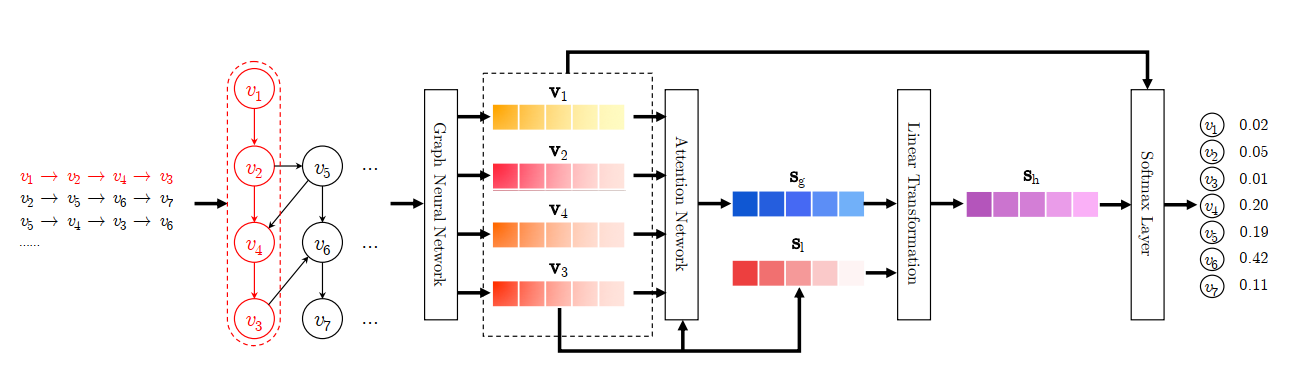# SRGNN¶

## Introduction¶

[paper]

Title: Session-based Recommendation with Graph Neural Networks

Authors: Shu Wu, Yuyuan Tang, Yanqiao Zhu, Liang Wang, Xing Xie, Tieniu Tan

Abstract: The problem of session-based recommendation aims to predict user actions based on anonymous sessions. Previous methods model a session as a sequence and estimate user representations besides item representations to make recommendations. Though achieved promising results, they are insufficient to obtain accurate user vectors in sessions and neglect complex transitions of items. To obtain accurate item embedding and take complex transitions of items into account, we propose a novel method, i.e. Session-based Recommendation with Graph Neural Networks, SR-GNN for brevity. In the proposed method, session sequences are modeled as graphstructured data. Based on the session graph, GNN can capture complex transitions of items, which are difficult to be revealed by previous conventional sequential methods. Each session is then represented as the composition of the global preference and the current interest of that session using an attention network. Extensive experiments conducted on two real datasets show that SR-GNN evidently outperforms the state-of-the-art session-based recommendation methods consistently.## Running with RecBole¶

Model Hyper-Parameters:

• `embedding_size (int)` : The embedding size of items. Defaults to `64`.

• `step (int)` : The number of layers in GNN. Defaults to `1`.

• `loss_type (str)` : The type of loss function. If it is set to `'CE'`, the training task is regarded as a multi-classification task and the target item is the ground truth. In this way, negative sampling is not needed. If it is set to `'BPR'`, the training task will be optimized in the pair-wise way, which maximizes the difference between the positive item and the negative one. In this way, negative sampling is necessary, such as setting `--train_neg_sample_args="{'distribution': 'uniform', 'sample_num': 1}"`. Defaults to `'CE'`. Range in `['BPR', 'CE']`.

A Running Example:

Write the following code to a python file, such as run.py

```from recbole.quick_start import run_recbole

parameter_dict = {
'train_neg_sample_args': None,
}
run_recbole(model='SRGNN', dataset='ml-100k', config_dict=parameter_dict)
```

And then:

```python run.py
```

## Tuning Hyper Parameters¶

If you want to use `HyperTuning` to tune hyper parameters of this model, you can copy the following settings and name it as `hyper.test`.

```learning_rate choice [0.01,0.005,0.001,0.0005,0.0001]
step choice [1, 2]
```

Note that we just provide these hyper parameter ranges for reference only, and we can not guarantee that they are the optimal range of this model.

Then, with the source code of RecBole (you can download it from GitHub), you can run the `run_hyper.py` to tuning:

```python run_hyper.py --model=[model_name] --dataset=[dataset_name] --config_files=[config_files_path] --params_file=hyper.test
```

For more details about Parameter Tuning, refer to Parameter Tuning.

If you want to change parameters, dataset or evaluation settings, take a look at